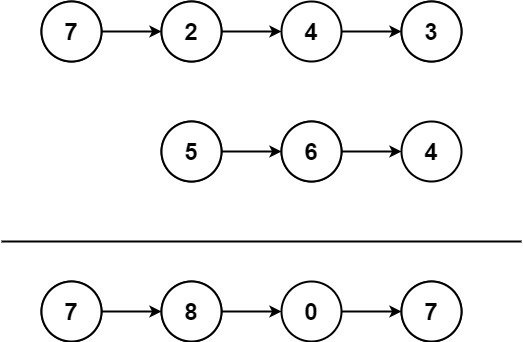# GeetCode Hub

You are given two non-empty linked lists representing two non-negative integers. The most significant digit comes first and each of their nodes contains a single digit. Add the two numbers and return the sum as a linked list.

You may assume the two numbers do not contain any leading zero, except the number 0 itself.

Example 1:```Input: l1 = [7,2,4,3], l2 = [5,6,4]
Output: [7,8,0,7]
```

Example 2:

```Input: l1 = [2,4,3], l2 = [5,6,4]
Output: [8,0,7]
```

Example 3:

```Input: l1 = , l2 = 
Output: 
```

Constraints:

• The number of nodes in each linked list is in the range `[1, 100]`.
• `0 <= Node.val <= 9`
• It is guaranteed that the list represents a number that does not have leading zeros.

Follow up: Could you solve it without reversing the input lists?

# Definition for singly-linked list. # class ListNode: # def __init__(self, val=0, next=None): # self.val = val # self.next = next class Solution: def addTwoNumbers(self, l1: ListNode, l2: ListNode) -> ListNode: